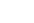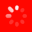The No.1 Website for Pro Audio
Search This Thread  Search This Forum  Search Reviews  Search Gear Database  Search Gear for sale  Search Gearslutz Go Advanced
Gear Nut

Sinc function confusion

Hey.
Recently i've started to dig deep into the aspects of digital audio.
The last few days iam reading some interesting papers at lavry's site.
At some point lavry is doing some tests with sinc functions.
I didn't have a clue what a sinc function was so i searched at wiki but things
got even more confusing!Wiki has a bunch of mathematical formulas as an explanation.I don't understand ****.
So if somenone have the patience to enligthten me with a slight simpler approach so i could move on i would be more than grateful.
Gear Head
The sinc function is defined as f(x)=sin(x)/x with sinc(0) defined as one.

The reason sinc functions are important in digital audio is because sinc functions are used to reconstruct a continuous bandlimited signal from its sample points. In particular, the reconstruction is simply the sum of shifted sinc functions each weighted by the sample point values.
Gear Addict

One problem I have when people quote Nyquist's theorem, is that it only applies when DA conversion is done using the sinc function. No real-world DA converters use the sinc function.
Gear Guru

Quote:
One problem I have when people quote Nyquist's theorem, is that it only applies when DA conversion is done using the sinc function. No real-world DA converters use the sinc function.

Think of it as a place-holder for the math...

-tINY

Gear Nut

Quote:
The sinc function is defined as f(x)=sin(x)/x with sinc(0) defined as one.

The reason sinc functions are important in digital audio is because sinc functions are used to reconstruct a continuous bandlimited signal from its sample points. In particular, the reconstruction is simply the sum of shifted sinc functions each weighted by the sample point values.
hallelujah!
I've started to understand now.
So AD converters use the sinc function process in real life?
Last but not least regarding the equation,i know that is a function equation
and f(x) is the input and the x after the equal is the output,but what the sin actually means?
Gear Nut

Quote:
One problem I have when people quote Nyquist's theorem, is that it only applies when DA conversion is done using the sinc function. No real-world DA converters use the sinc function.
What is the process in DA converters?
Lives for gear

Quote:
sine wave
Gear Guru

Quote:
hallelujah!
,but what the sin actually means?

SOH-CAH-TOA

opposite over hypotenuse...

-tINY

Gear Nut

I would have thought that.
Iam dumb!
Thank you for your time.
It's time for study
Gear Nut

Quote:

SOH-CAH-TOA

opposite over hypotenuse...

-tINY

Yes i got it!
But i have to get back to maths!
Thanks
Lives for gear

you can download this app.(wolfram cdf player) to view the linked page and use the interactive graphs.
sometimes i need a visual and the wolfram site is nice for that...
Wolfram Demonstrations Project
Gear Head
Quote:
One problem I have when people quote Nyquist's theorem, is that it only applies when DA conversion is done using the sinc function. No real-world DA converters use the sinc function.
I don’t understand why you have a problem describing sampling theory using sinc functions. That is the math of the theory. How to implement the math in practice is another issue. For example, you can implement the summing of shifted sinc functions using impulse samples feeding an ideal low pass filter. The math is the same. You can describe the filter in the time domain (i.e., impulse response) or the frequency domain (ideal cutoff filter). The impulse response of an ideal low pass filter is a sinc function.

Now in practice, you have to use approximations. Sinc functions are not practical (they theoretically go from –inf to +inf) and ideal low pass filters cannot be realized. There are also other practical issues including using flat-top samples with equalization compensation, oversampling to make the reconstruction filter simpler, etc… . But no matter how you implement it, the math involves sinc functions (or approximations to sinc functions).
Gear Addict

Quote:
I don’t understand why you have a problem describing sampling theory using sinc functions. That is the math of the theory. How to implement the math in practice is another issue. For example, you can implement the summing of shifted sinc functions using impulse samples feeding an ideal low pass filter. The math is the same. You can describe the filter in the time domain (i.e., impulse response) or the frequency domain (ideal cutoff filter). The impulse response of an ideal low pass filter is a sinc function.

Now in practice, you have to use approximations. Sinc functions are not practical (they theoretically go from –inf to +inf) and ideal low pass filters cannot be realized. There are also other practical issues including using flat-top samples with equalization compensation, oversampling to make the reconstruction filter simpler, etc… . But no matter how you implement it, the math involves sinc functions (or approximations to sinc functions).
You misunderstood my point, but answered it anyway. I have no problem with describing sampling theory using sinc functions, that would be silly. Nyquist's applies to ideal converters, you cannot apply it to non-ideal (ie real world) conversion with filters. This non-ideal conversion is why 44.1kHz was chosen for CDs, not 40kHz.

You may call it pedantry.
Lives for gear

Quote:
So AD converters use the sinc function process in real life?
Last but not least regarding the equation,i know that is a function equation
and f(x) is the input and the x after the equal is the output,but what the sin actually means?
Hi, mathematical functions are used for modeling the physical world -- they're convenient for working out the theory/predictions/etc -- but they are not physical objects.

The symbols used to describe functions work like this:

When the value of some number y depends on the value of some other number x, we say that y is a function of x. We express this in symbols like so:
y = f(x)
where f is some rule for determining the value of y, given x. You can think of f as a black box: you put in some number x and out comes another number y. For example, if the rule in my black box is "add one to whatever comes in", I could notate it as
f(x) = x + 1
Putting actual numbers in place of x would give you:
2 = f(1)
3 = f(2)
11 = f(10)
and so on. This is a very simple blackbox, but we can make them as complicated and fancy as we require. For example, this blackbox
f(x) = 1/(x^2 + 1)
maps every input to the reciprocal of its square. If we plot all the outputs on a grid, we get an elegant looking bell-shaped curve (with the cool ass name Witch of Agnesi). These graphs of functions are incredibly valuable because they let us "see" the behavior of a function over a whole range of inputs in one picture.

You've almost certainly seen the graph of the sine function, a smooth up-down wave-like curve (there's one in my avatar picture in red, along with its cousin the cosine, in blue). The sine function belongs to a special group of periodic functions (i.e., those that forever repeat a pattern) that are so useful they've been given their own names: while most functions are anonymously labeled f(x), the sine function is labeled sin(x).

What exactly is inside the blackbox of sin(x)? Unfortunately this doesn't have a simple answer -- it is a so-called transcendental function -- but rest assured it is well-defined. And it turns out that when you multiply sin(x) by x itself, you get an explosive curve that is damped toward the origin (the middle of the graph). When you divide sin(x) by x itself, you get the opposite graph: a big curve at the origin that's damped as it gets farther out. This behavior proves useful in modeling many things, so we've given it its own name: sinc(x).

That so very many phenomena in nature can be modeled by relatively simple mathematical functions is, I think, one of the great and beautiful mysteries of the universe.
Topic:

## Welcome to the Gearslutz Pro Audio Community!

###### Registration benefits include:
• The ability to reply to and create new discussions
• Access to members-only giveaways & competitions
• Interact with VIP industry experts in our guest Q&As
• Access to members-only sub forum discussions
• Access to members-only Chat Room
• Get INSTANT ACCESS to the world's best private pro audio Classifieds for only USD \$20/year
• Promote your eBay auctions and Reverb.com listings for free
• Remove this message!
You need an account to post a reply. Create a username and password below and an account will be created and your post entered.Slide to join now Processing…
Thread Tools
Search this Thread
Search this Thread:

Advanced Search
Forum Jump
Forum Jump